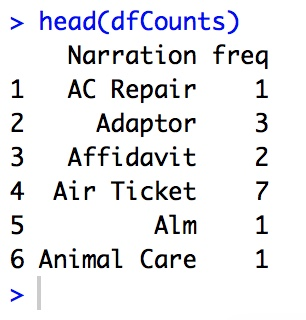Technical

# WordCloud

Creating WordCloud with R Language is rather easy. I demonstrate how to create WordCloud for structured data and how to create the same for unstructured data.

First we create a WordCloud using structured data. I track my expenses on a minute-by-minute basis. The data contains the narration for the expense, the category for the expense, the amount of the expense and the date of the expense. We create the WordCloud for the Narration column first.

To start, first include the following libraries.

```if("plyr" %in% rownames(installed.packages()) == FALSE) {install.packages("plyr")}
library(plyr)

if("wordcloud" %in% rownames(installed.packages()) == FALSE) {install.packages("wordcloud")}
library(wordcloud)<span id="mce_SELREST_start" style="overflow: hidden; line-height: 0;"></span>
```

Next, we upload the csv file containing the data.

```df <- read.csv("Expenses.csv", sep = ",")
```

To see the data, use the head command.

```head(df)
```From this data, we need to build the frequency table. For this, we can use the plyr package. We store the frequencies in a data frame named dfCounts.

```dfCounts <- count(df, 'Narration')
```

To see the frequency table, use the head command.

```head(dfCounts)
```Now we are ready to build the WordCloud. Use the wordcloud function from the wordcloud package.

```wordcloud(words = dfCounts\$Narration, freq = dfCounts\$freq, min.freq = 1,
max.words=250, colors=brewer.pal(8, "Dark2"),
random.order = TRUE )
```

The output is as follows.By setting the random.order parameter to false, the output would be as shown below.

```wordcloud(words = dfCounts\$Narration, freq = dfCounts\$freq, min.freq = 1,
max.words=250, colors=brewer.pal(8, "Dark2"),
random.order = FALSE )
```You can see that I buy Tea a number of times. Also, I need eating in Hotels and invest quite frequently in Mutual Fund.

## Now, let us see how we can build a WordCloud for unstructured data.

For this, we will require Text Files. We can use any number of Text Files as long as we store them on a single directory on the machine. However, for this we will only use one file and that is my resume.

As before, we first include the libraries.

```if("tm" %in% rownames(installed.packages()) == FALSE) {install.packages("tm")}
library(tm)

if("wordcloud" %in% rownames(installed.packages()) == FALSE) {install.packages("wordcloud")}
library(wordcloud)
```

Next, we need to upload the files. We can see the contents of the loaded file(s) using the inspect command.

```dirPath <- "/Users/parthamajumdar/Documents/Education/Watson/Data"

resumeData <- Corpus(DirSource(dirPath))

inspect(resumeData)
```

Next we need to clean the documents.

```# Convert the text to lower case
resumeData <- tm_map(resumeData, content_transformer(tolower))

# Remove numbers
resumeData <- tm_map(resumeData, removeNumbers)

# Remove english common stopwords
resumeData <- tm_map(resumeData, removeWords, stopwords("english"))

# Remove your own stop word
# specify your stopwords as a character vector
resumeData <- tm_map(resumeData, removeWords, c("pvt", "ltd", "jan", "feb", "mar", "apr", "may", "jun", "jul", "aug", "sep", "oct", "nov", "dec"))

# Remove punctuations
resumeData <- tm_map(resumeData, removePunctuation)

# Eliminate extra white spaces
resumeData <- tm_map(resumeData, stripWhitespace)
```

After that we need to form the TermDocumentMatrix and create the word frequencies.

```# Create a Term Document Matrix
dtm <- TermDocumentMatrix(resumeData)

# Matrix transformation
m <- as.matrix(dtm)

#Sort it to show the most frequent words
v <- sort(rowSums(m),decreasing=TRUE)

#transform to a data frame
d <- data.frame(word = names(v),freq=v)
```

Now, we are ready to form the WordCloud.

```wordcloud(words = d\$word, freq = d\$freq, min.freq = 1,
max.words=250, colors=brewer.pal(8, "Dark2"),
random.order = FALSE )
```This site uses Akismet to reduce spam. Learn how your comment data is processed.